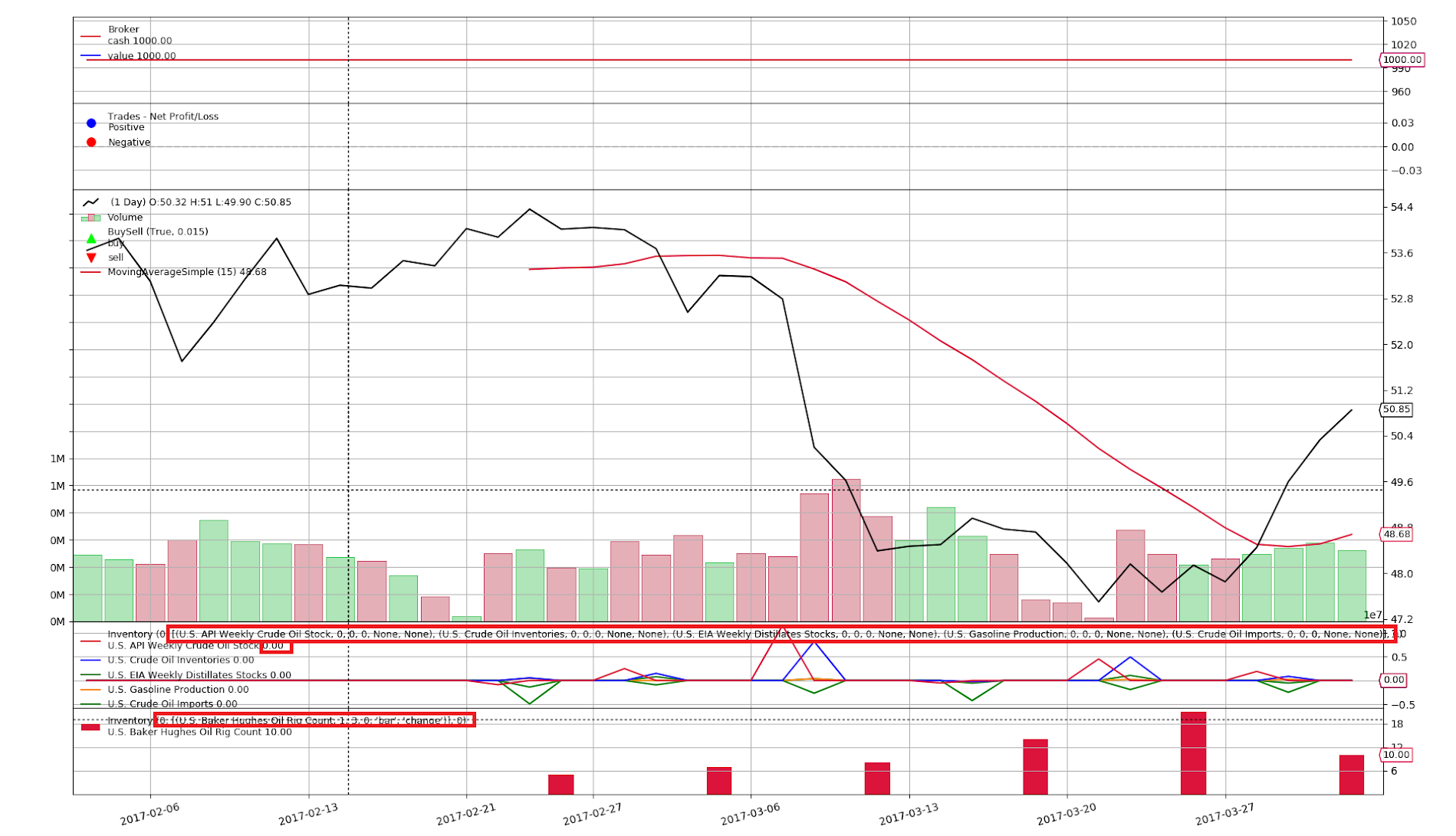For code/output blocks: Use ``` (aka backtick or grave accent) in a single line before and after the block. See: http://commonmark.org/help/

• Imagine indicator that loads news from data sources list, which was passed by `params`. List length is variable.

Now how to add dynamically lines to indicator?

• You cannot dynamically add lines. Not because it cannot be done, because the code filling the lines would also have to be changed dynamically and the number of lines would change along the datetime axis.

• Ok then is it possible to hide and rename lines? Thus one can add 10 lines with pretty generic names, then hide not necessary one and rename visible?

• It seems you are concerned about plotting those lines because you specifically say `hide` and thus the assumption that the same applies to `rename`.

You can change the `plotlines` information for any line.

See Docs - Plotting and specifically the section Line specific plotting options. The `plotlines` information can be changed in a live instance.

• From `Stochastic` for example

``````    lines = ('percK', 'percD',)
...

plotlines = dict(percD=dict(_name='%D', ls='--'),
percK=dict(_name='%K'))
``````

Used because `%` is not an allowed character in identifiers. And from the `DrawDown` observer

``````    lines = ('drawdown', 'maxdrawdown',)

...

plotlines = dict(maxdrawdown=dict(_plotskip=True,))
``````

• Here and in other indicators plotlines are static defined. Is `_plotinit` right place to redefine it?

• Definition only happens when the class is created. At any other time including `_plotinit`, things happen in instances and there you can only change the values of the already defined things.

• Got it too.
Last question: how to reach exact plotline in loop, not calling it by name? I.e. not like this

``````self.plotlines.<line_name>._name = 'new'
``````

Studying `AutoInfoClass`...

• You cannot. What you can do is name the lines sequentially and then access them sequentially.

• Could you provide code snippet please?

• Standard python ...

``````lines = ('line1', ..., 'lineX')
plotlines = dict(
line1=dict(...),
...
lineX = dict(...),
)
``````
``````for i in range(1, X + 1):
plotline = getattr(self.plotlines, 'line' + i)
# do something with the plotline
``````

• Thank you for all replies and code snippets.

Here is the beauty:I suggest you to add options to hide some text to make data cleaner - see red rectangles on image:

1. Indicator parameters list;
2. Line last value.

• Whatever is shown after the name of the indicator can be controlled. See the following excerpt from `RSI`:

``````    def _plotlabel(self):
plabels = [self.p.period]
plabels += [self.p.movav] * self.p.notdefault('movav')
return plabels
``````

The method returns a list of strings, with one special provision to use the name of some classes as the text label to automatically support using other indicators.

• Thanks!

It's much cleaner now:Dreaming of `disable showing last value` feature.

• Both `_plotlabel` and `_plotinit` have now been documented under Docs - Plotting

• Am I missing something or there is not option to reach (cancel) this code in `Plot_OldSync.plotind()`?

``````        if not math.isnan(lplot[-1]):
label += ' %.2f' % lplot[-1]``````

• backtrader is not the 1st platform to plot ... and a common pattern is to plot the last value of the indicator. So far no option to disable it.

• This was anyhow not a breaking change, so the `development` branch contains now

• Control over the last value after the name of the object

• Global: `linevalues` (as option to `plot` or in a `PlotScheme` instance)
• Per-object: `plotinfo.plotlinevalues`
• Per-line (for indicators/observers): `_plotvalue`
• Control over the right hand side tag on lines:

• Global: `valuetags` (as option to `plot` or in a `PlotScheme` instance)
• Per-object: `plotinfo.plotvaluetags`
• Per-line (for indicators/observers): `_plotvaluetag`

• Part of the small release 1.9.38.116 and added to the plotting documentation

• In my particular case

``````line._plotvalue = False
``````

helps.

Thanks for flexible solution!

});